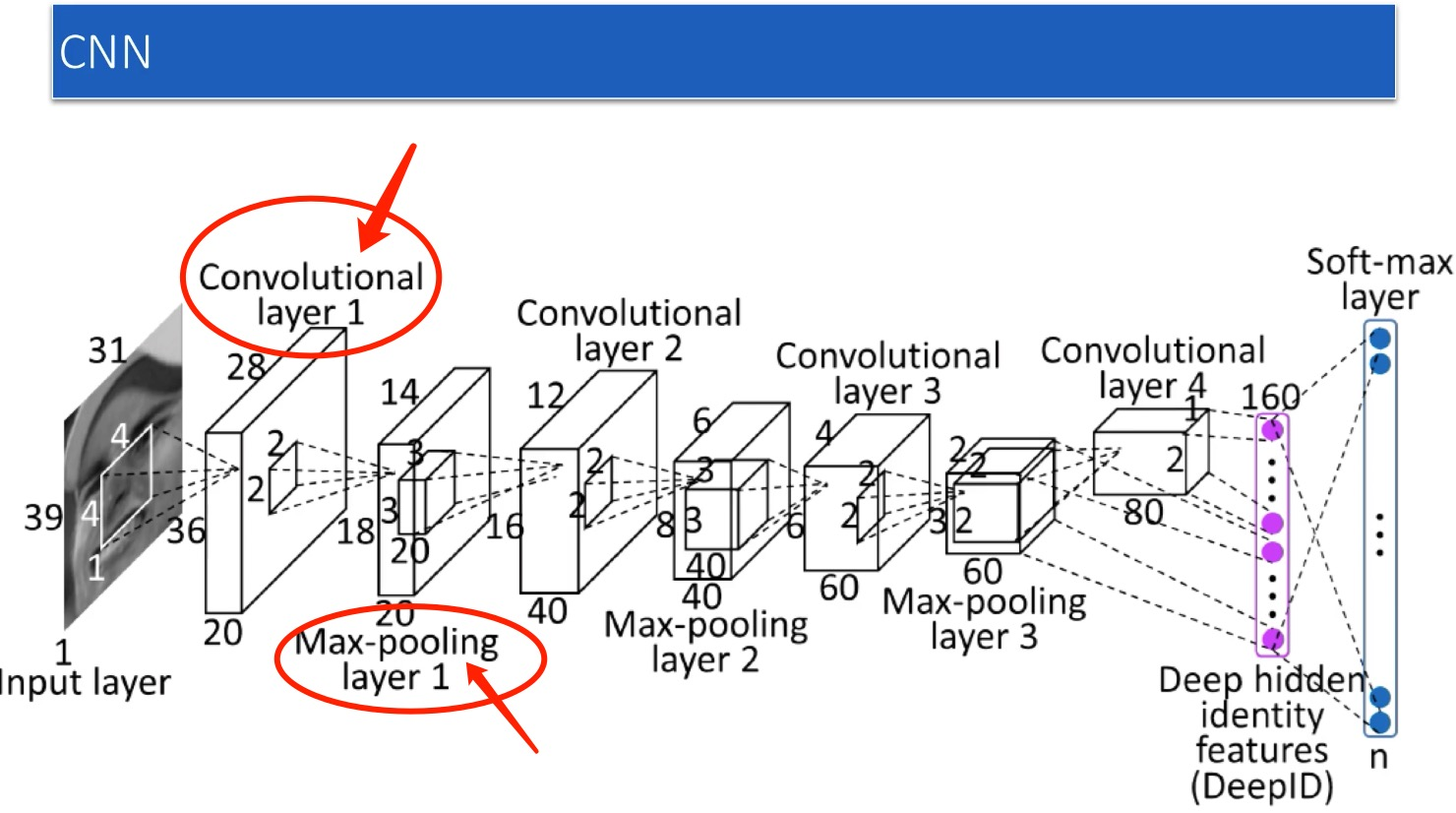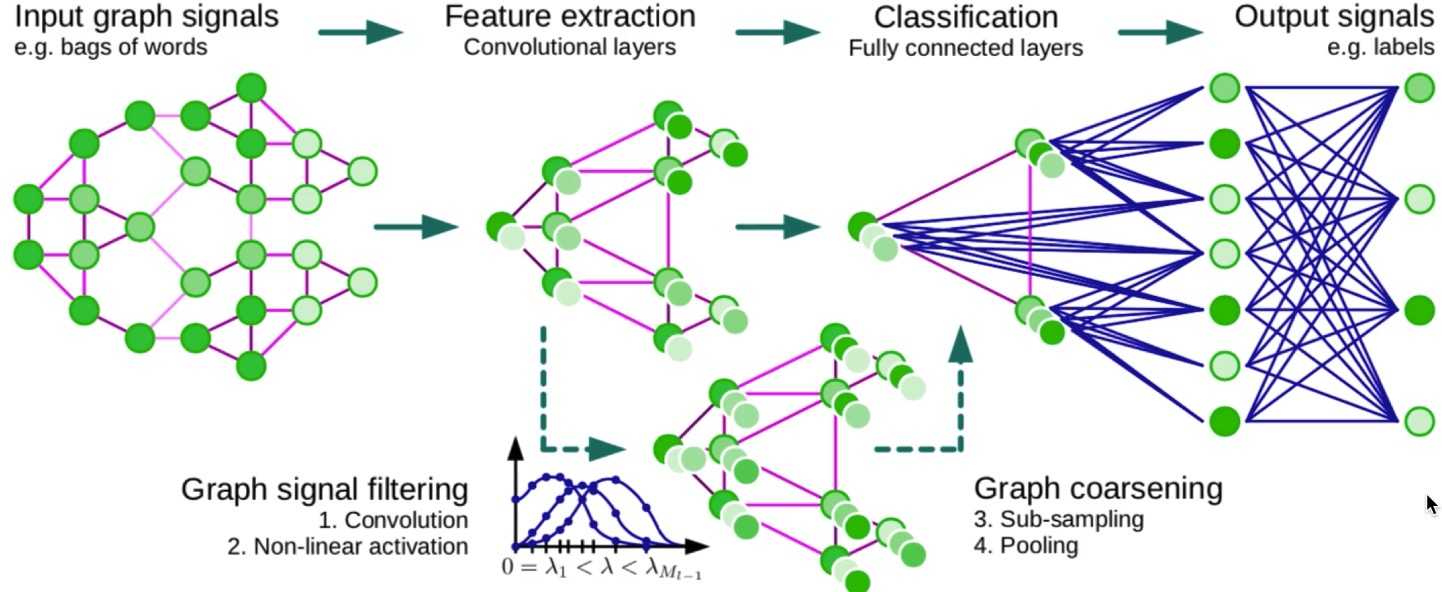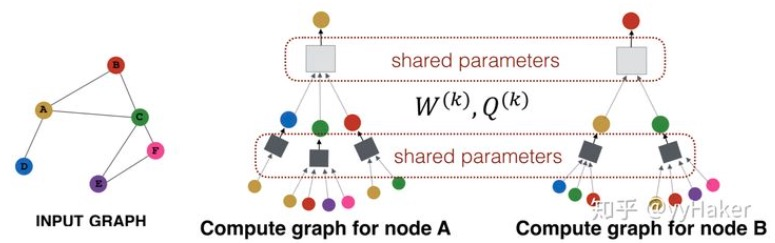# 概述

• 图、图神经网络的基本概念
• 图上如何做卷积和池化
• 卷积的两种：频谱卷积和空间卷积
• 常见的图卷积网络（注意不是图神经网络，那个范畴更大，我用不到，暂时也不做深入研究）
• 给出我收集的资料

## 图

$G=(V,E,W)$

• V 是节点（vertex）集合，其中节点用一个d维向量表示，$n=|V|$
• X 是节点的向量矩阵，就列就是一个节点的表示，$X \in R^{n \times d}$
• E 是边（edge）集合
• W 是邻接矩阵（方阵），矩阵里的值，就是边的权重，$W \in R^{n \times n}$

## 图神经网络的用途

• 一个网络，我最终给这个网络一个分类
• 一个网络，我对网路中的每个节点进行分类（社交网络中的标签分类）
• 一个网络，我通过局部网络的结构（已知的标注网络）学习出另外部分的网络结构（如预测一个节点和别人的链接情况）（是否用来做OCR布局分析？）
• 还可以和GAN结合，用于生成一个网络结构（分子制药生成分子式）

# 图卷积

## 类比CNNCNN只适合欧式空间，比如图像。而基于图（Graph）GNN更通用，适合非欧空间（啥叫欧式空间，就是支持距离公式的空间。） 可是，现在，我还是需要做卷积、池化，我改如何做？

## 先说说卷积• 对应上图：$f(\tau)$就是右侧那个下降函数，$g(x-\tau)$是方波函数，而卷积结果（是个以时间$\t$为变量的函数）是左侧那个上升函数
• $h(t)$是一个以$t$为变量的函数，是的，他是个时间函数
• 设想某个$t=t_0$，$h(t_0)$就是那上图中黄色面积
• 这个面积是一个积分，是$\tau$从$-\infty ~ +\infty$的积分，上图上，就是黄色的面积

# 谱域卷积

## 初始概念

【1、图上的拉普拉斯分解】

$L=D - W$

• W上面提到了，就是边上的权重，是一个对称方阵，$W \in R^{n \times n}$
• D呢？我看很多文章是都说图的节点的度的对角阵，但是实际上D的定义是：$D_{ij}= \sum_j W_{ij}$。

$L = U \begin{pmatrix} \lambda_1 & & \\ & \ddots & \\ & & \lambda_n \end{pmatrix} U^T$

【2、图上的频域傅里叶变换】• $f$是Graph上的N维向量
• $f(i)$就是第$i$个节点的向量表达
• $u_l(i)$就是第$l$个特征向量的第$i$维度的值
• $\hat f(\lambda_l)$，就是到了频域后，这个频率$\lambda_i$对应的值。

【3、频域上的卷积】

$\hat f \odot \hat h = U^T f \odot U^T h$

$\odot$ 是Hamada积，表示逐点相乘

$(f*h)_G = U( (U^T f) \odot (U^T h))$

【5、迭代起来】

• 多参数，我觉得好像是没有，这个网络里，就一组参数，就是$g_\theta$
• 多层网络，这个有，就是学完后，每个节点上的隐含表达更新了，那下一轮再重复这个过程，我理解就算是“下一层”了
• 如何更新参数？还是梯度下降啊，那也必须得有损失函数loss啊。这个，肯定是要依赖于下游任务了，比如节点分类，节点关系预测啥的。

$h$是卷积核，对吧，长啥样？其实不用关心，因为卷积，不像之前的CNN（是在时域上做的），现在变到了频域上去了，所以你现在应该关心的是，频域上的卷积核长啥样？

$\begin{pmatrix} \theta_1 & & \\ & \ddots & \\ & & \theta_n \end{pmatrix}$

【4、可以深度学习了】

$(f*h)_G = U( g_\theta \odot (U^T h))$

$(f*h)_G = \sigma( U( g_\theta \odot (U^T h)))$

【它的问题】

• 他得要求这个Graph节点数不能变，因为上述公式中的$U$是特征向量，你的节点一变，拉普拉斯矩阵就变了，你的$U$就得重新求了
• 你学的参数$g_\theta$，是个N维度，跟你节点数一样的啊，你节点数一遍，参数个数都得变啊
• 还有，这个卷积核$g_\theta$是跟整个图相关的，原因是它和整个图的特征分解相关啊，这样，他也不是局部化的，你说非局部化有啥不好？我理解是，你没法像传统CNN那样，通过一个小卷积核，通过局部的卷积操作，可以学出整个图的特征。

## 改进

$g_\theta = \begin{pmatrix} \theta_1 & & \\ & \ddots & \\ & & \theta_n \end{pmatrix}$

ChebNet，来了个神奇的操作，把$g_\theta$变成了关于特征值n次$\lambda_i$的多项式：$g_\theta(\Lambda)$

$g_\theta(\Lambda) = \begin{pmatrix} \sum_{j=0}^K \theta_j \lambda_1^j & & \\ & \ddots & \\ & & \sum_{j=0}^K \theta_j \lambda_n^j \end{pmatrix}$

$(g_\theta * x)=> \\ U\sum_{j=0}^K \theta_j \Lambda^j U^T x \\ = \sum_{j=0}^K \theta_j (U \Lambda^j U^T) x \\ = \sum_{j=0}^K \theta_j (U \Lambda U^T)^j x \\ = \sum_{j=0}^K \theta_j L^j x \\$

• 特征分解、特征向量求取麻烦
• 卷积核是全局相关的

## ChebnetNet

【参考】

• 既然上面创新性的用一个特征值n次多项式替换了参数，

ChebNet，来了个神奇的操作，把$g_\theta$变成了关于特征值n次$\lambda_i$的多项式：$g_\theta(\Lambda)$

• 得降维吧！过去图多大，参数$g_\theta$就多少维度，不好！现在咱们得想办法给他降到一个固定的低维。
• 别全局相关，最好只考虑局部的特征就能学出全图来，这样，我不用全图信息，也能学出全图，比如通过采样啥的

### 切比雪夫多项式

$cos(\theta) = cos(\theta) \\ cos(2\theta) = 2cos^2(\theta) -1 \\ cos(3\theta) = 4cos^3(\theta) - 3cos(\theta) \\ cos(4\theta) = 8cos^4(\theta) - 8cos^2(\theta) + 1$

$g(x) = cos(\theta) = cos( arc cos(x)) \\ g(x) = cos(2\theta) = cos( 2arc cos(x)) \\ g(x) = cos(3\theta) = cos( 3arc cos(x)) \\ g(x) = cos(4\theta) = cos( 4arc cos(x))$

Chebyshev多项式的递推公式: $g_{n+1}(x) = 2xg_n(x) - g_{n-1}(x)$

$p(x) = \sum_{n = 0}^{3} a_nT_n = a_0 T_0(x)+a_1 T_1(x)+a_2 T_2(x)+a_3 T_3(x)$，

$p(x)$是我们造出来的最高项为3次方的多项式，去拟合题目中给的4个点$(1,1),(2,3),(3,5),(4,4)$，把$x$带入进去，就可以递归的推出$T_i(x)$，结果$y$你也知道，剩下的就是解一个线性方程，求出这些待定系数$a_i$了。

### ChebNet

$g_\theta(\Lambda)=\sum_{j=0}^K \theta_j \Lambda^j \rightarrow \sum_{j=0}^K \theta_j T_k(\hat \Lambda)$

ChebNet，来了个神奇的操作，把$g_\theta$变成了关于特征值n次$\lambda_i$的多项式：$g_\theta(\Lambda)$

$g_\theta(\Lambda) = \begin{pmatrix} \sum_{j=0}^K \theta_j \lambda_1^j & & \\ & \ddots & \\ & & \sum_{j=0}^K \theta_j \lambda_n^j \end{pmatrix}$

$g(\lambda_1) = \theta_1 \lambda_1 + \theta_2 \lambda_1^2 + ... + \theta_K \lambda_1^K \rightarrow \\ = \theta_1 T_0(\lambda_1) + \theta_2 T_0(\lambda_1^2) + ... + \theta_K T_0(\lambda_1^K) \\ T_i(\lambda_1) 就是切比雪夫递归式项$

$(g_\theta * x) \\ = U \sum_{j=0}^K \theta_j \Lambda^j U^T x \\ \approx U \sum_{j=0}^K \theta_j T_j(\Lambda^j) U^T x \\ 因为Chebyshev多项式作用在对角矩阵上，不会影响矩阵运算\\ 那就改变一下运算顺序，先把矩阵运算放进去 \\ = \sum_{j=0}^K \theta_j T_j (U (\Lambda^j) U^T) x \\ = \sum_{j=0}^K \theta_j T_j (L) x \\$

OK! 现在再观察这个式子：$(g_\theta * x)=\sum_{j=0}^K \theta_j T_j (L) x$，$L$是已知的，而$T_j(L)$，也可以递归推出，那剩下的就是需要梯度下降求解的参数$\theta_j$了，而且，计算复杂度也降下来了，只要递归推一下$T_j$既可以，是$O(n)$复杂度的了。

$\hat L = \frac{2}{\lambda_{max}} L - I$

Chebyshev多项式的递推公式: $g_{n+1}(x) = 2xg_n(x) - g_{n-1}(x)$

$T_0(\hat L) = I \\ T_1(\hat L) = \hat L \\ T_j(\hat L) = 2T_{j-1}(\hat L) - T_{j-2}(\hat L)$

• 我们要模仿CNN的卷积，为何我们要做卷积呢？我们是为了做不同信号的滤波。
• 可是图网络不具备空间不变形，所以呢，只好把卷积核设计到了频域里。参照傅里叶变换，我们用拉普拉斯矩阵的特征向量，当我们的正交基，来做变换。
• 这样下来，需要做特征值分解，且，卷积核不具备局部性，只好改进。
• 改进的方法是先生生憋出一个基于特征值的多项式，来替换频域的参数$\theta$
• 然后，在进一步，用切比雪夫多项式，来逼近这个特征值的多项式

## GCN

$(g_\theta * x) = \\ \sum_{j=0}^K \theta_j T_j (L) x \\ \approx \theta_0 x + \theta_1(\hat L) \\ = \theta_0 x + \theta_1(\hat L) \\ = \theta_0 x + (\frac{2}{\lambda_{max}} L - I) x \\ \lambda_{max} \leqslant 2(这个我也没深究，是可以证明的) \\ \approx \theta_0 x + \theta_1(L - I) x \\ = \theta_0 x + \theta_1(L - I) x \\ = \theta_0 x + \theta_1( D^{- \frac{1}{2}} W D^{-\frac{1}{2}} ) x \\ 这个是拉普拉斯矩阵的对称归一化的拉普拉斯矩阵 \\ 然后，再简化一下，令\theta_0 = -\theta_1，上式继续简化，\\ = \theta(I_n + D^{-\frac{1}{2}} W D^{-\frac{1}{2}} ) \\ 由于谱半径[0,2]太大，归一化一下（为何做这步？没搞明白）\\ = \hat D^{-\frac{1}{2}} \hat W \hat D^{-\frac{1}{2}}\\ 其中，\hat W = W + I_n, \hat D_{ij} = \sum_j \hat W_{ij}$

$x$就是graph上对应每个节点的feature构成的向量，$x=(x_1,x_2,…,x_n)$ ​，这里暂时对每个节点都使用标量，相当于channel为1。

$\underbrace{Z}_{\mathbb R^{N \times F}} = ( \underbrace{ \hat D^{-\frac{1}{2}} \hat W \hat D^{-\frac{1}{2}}}_{\mathbb R^{N \times N}} ) \underbrace{X}_{\mathbb R^{N \times C}} \underbrace{\Theta}_{\mathbb R^{C \times F}}$

GCN已经把谱域家族荣耀到极致了，但是，他仍然是不完美的：

GCN的缺点也是很显然易见的:第一，GCN需要将整个图放到内存和显存，这将非常耗内存和显存，处理不了大图；第二，GCN在训练时需要知道整个图的结构信息(包括待预测的节点)

# 空域卷积

## GraphSAGE

Inductive（归纳） learning和Transductive（直推） learning的概念？就讨厌玩概念，Inductive，就是从局部的图推演出整体的图特性，就是用图上某些有限的节点和关系，学习出整个图上泛化特性。而Transductive就得用整个图的信息才可以，前面的那一堆谱方法，都是用所有的节点，即使是最简化的GCN，也需要所有的节点信息（$\underbrace{X}_{\mathbb R^{N \times C}}$ 里的N，就表明是所有的节点）。

GrachSAGE（Graph Sample and Aggregate）就是为了解决GCN的上面说的两个问题：GraphSAGE是这样玩的：对每一个节点，随机采样他的K跳近邻，采样出一颗子图（其实是个树吧?），然后使用一个聚合函数（这个函数得设计），分K层依次聚合，就跟消息传递似的，一直从边缘传播到中心，更新这个节点的embeding表示。

【咋采样？】

【这里参数是啥？】【咋聚合？参数呢？】

【损失函数】

【GraphSAGE得到了啥】

【GraphSAGE的缺点】

GraphSAGE也有一些缺点，每个节点那么多邻居，GraphSAGE的采样没有考虑到不同邻居节点的重要性不同，而且聚合计算的时候邻居节点的重要性和当前节点也是不同的。这个就需要下面介绍的GAT（Graph Attention Networks）来解决喽。

## GATpytorch的那个项目

【目前看过的】

【待学习的】